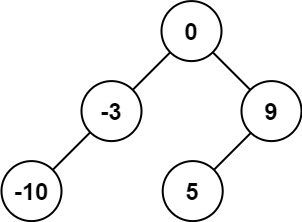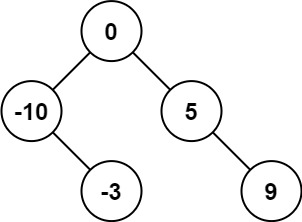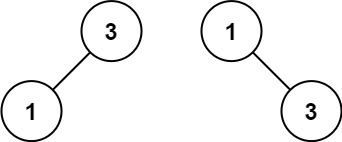# 108. Convert Sorted Array to Binary Search Tree / Easy

Given an integer array nums where the elements are sorted in ascending order, convert it to a height-balanced binary search tree.

A height-balanced binary tree is a binary tree in which the depth of the two subtrees of every node never differs by more than one.

## Example 1:Input: nums = [-10,-3,0,5,9]
Output: [0,-3,9,-10,null,5]
Explanation: [0,-10,5,null,-3,null,9] is also accepted:## Example 2:Input: nums = [1,3]
Output: [3,1]
Explanation: [1,null,3] and [3,1] are both height-balanced BSTs.

## Constraints:

• 1 <= nums.length <= 10^4
• -10^4 <= nums[i] <= 10^4
• nums is sorted in a strictly increasing order.

# Solution: Recursive

## 效能

### Complexity

• Time Complexity: O(N)
• Space Complexity: O(logN)

### LeetCode Result

• Runtime: 21 ms
• Memory Usage: 21.5 MB